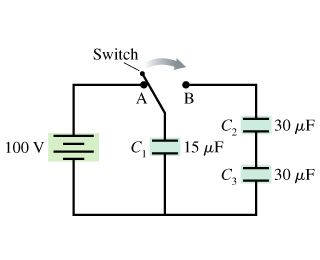# Problem: Initially, the switch in the figure is in position A and capacitors C2 and C3 are uncharged. Then the switch is flipped to position B. (Figure 1)A) Afterward, what is the charge on C1 capacitor? (I tried doing 15 times 100 for 1500 uC but it won't get accepted for some reason. Help!!)B) Afterward, what is the potential difference across C1 capacitor?C) Afterward, what is the charge on C2 capacitor?D) Afterward, what is the potential difference across C2 capacitor?E) Afterward, what is the charge on C3 capacitor?F) Afterward, what is the potential difference across C3 capacitor?

###### FREE Expert Solution

For 2 capacitors in series, the equivalent capacitance is:

$\overline{){{\mathbf{C}}}_{\mathbf{e}\mathbf{q}}{\mathbf{=}}\frac{{\mathbf{C}}_{\mathbf{1}}{\mathbf{C}}_{\mathbf{2}}}{{\mathbf{C}}_{\mathbf{1}}\mathbf{+}{\mathbf{C}}_{\mathbf{2}}}}$

For capacitors in parallel, the equivalent capacitance is:

$\overline{){{\mathbf{C}}}_{\mathbf{e}\mathbf{q}}{\mathbf{=}}{{\mathbf{C}}}_{{\mathbf{1}}}{\mathbf{+}}{{\mathbf{C}}}_{{\mathbf{2}}}{\mathbf{+}}{\mathbf{.}}{\mathbf{.}}{\mathbf{.}}{\mathbf{+}}{{\mathbf{C}}}_{{\mathbf{n}}}}$

The charge stored by a capacitor:

$\overline{){\mathbf{Q}}{\mathbf{=}}{\mathbf{C}}{\mathbf{V}}}$

A)

C2 and C3 are in series:

C23 = (30)(30)/(30 + 30) = 15 μF

The initial charge Q0 = C1V = (15 × 10-6)(100) = 1.5 × 10-3 C

This charge will distribute equally among capacitor C1 and C23.

81% (434 ratings)###### Problem Details

Initially, the switch in the figure is in position A and capacitors C2 and C3 are uncharged. Then the switch is flipped to position B. (Figure 1)A) Afterward, what is the charge on C1 capacitor? (I tried doing 15 times 100 for 1500 uC but it won't get accepted for some reason. Help!!)
B) Afterward, what is the potential difference across C1 capacitor?
C) Afterward, what is the charge on C2 capacitor?
D) Afterward, what is the potential difference across C2 capacitor?
E) Afterward, what is the charge on C3 capacitor?
F) Afterward, what is the potential difference across C3 capacitor?